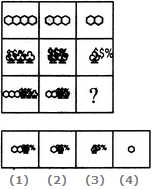# Non Verbal Reasoning - Figure Matrix - Discussion

### Discussion :: Figure Matrix - Section 1 (Q.No.19)

In each of the following questions, find out which of the answer figures (1), (2), (3) and (4) completes the figure matrix ?

19.

Select a suitable figure from the four alternatives that would complete the figure matrix.[A]. 1 [B]. 2 [C]. 3 [D]. 4

Explanation:

In each column, the third figure (lowermost figure) contains one less number of hexagons as the first figure (uppermost figure) and the same number of trees as the second figure (middle figure).

 Prateek Aggarwal said: (Aug 26, 2016) The trees and the hexagons are not too clear in the figure. So, I can't able to find the solution.